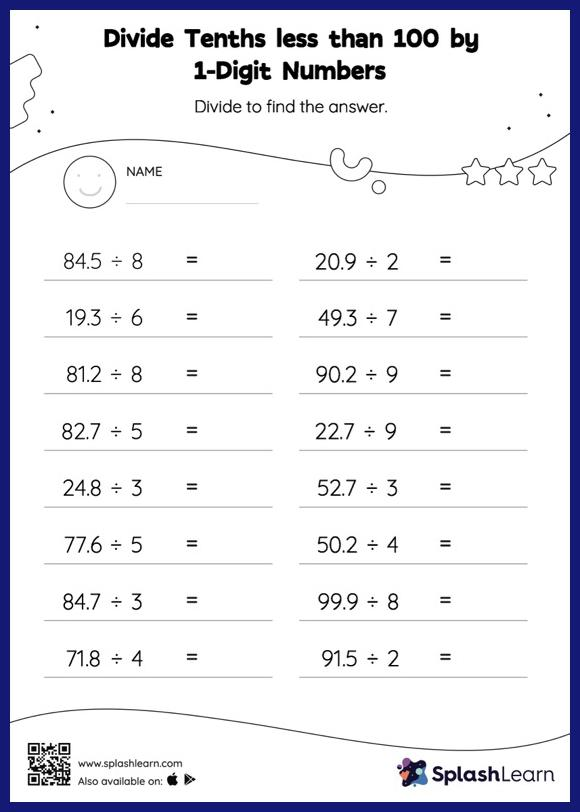# Divide Tenths less than 100 by 1-Digit Numbers with Remainder: Horizontal Division Worksheet

Home > Divide Tenths less than 100 by 1-Digit Numbers with Remainder: Horizontal DivisionThis divide tenths less than 100 by 1-digit numbers with remainder worksheet consists of tasks to help your young mathematician develop fluency with division. This worksheet is about practicing with the horizontal format in which numbers are written side by side. To develop flexibility with numbers and operations, students need to have enough practice in this format and not just rely on the vertical/column method.